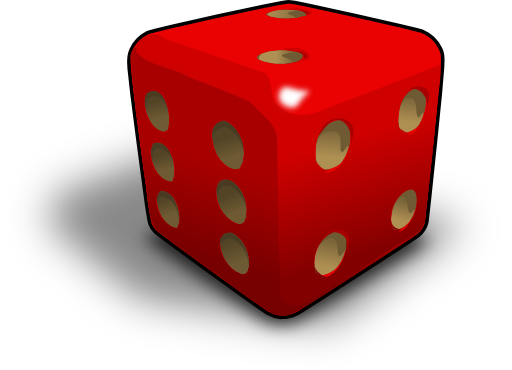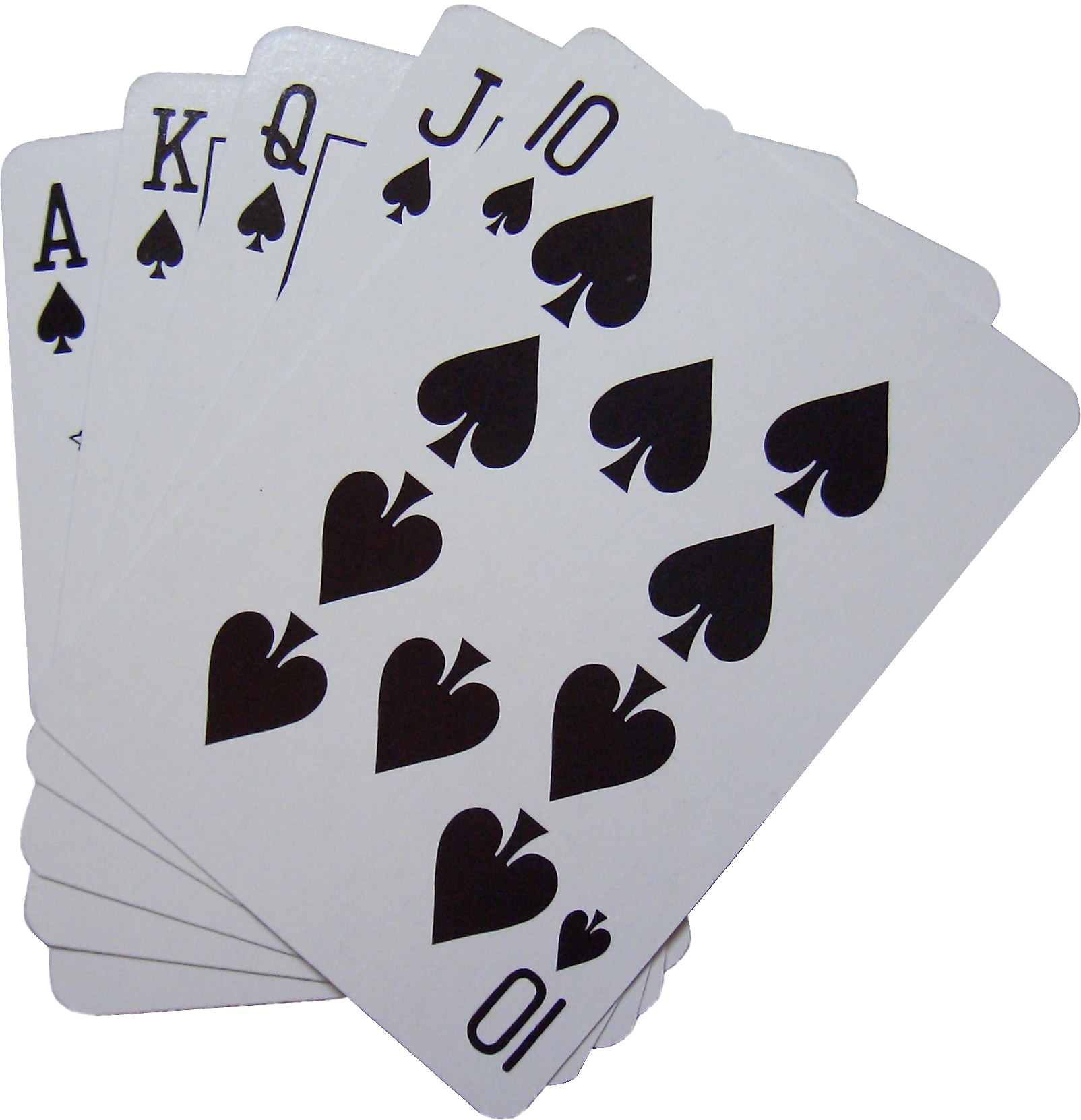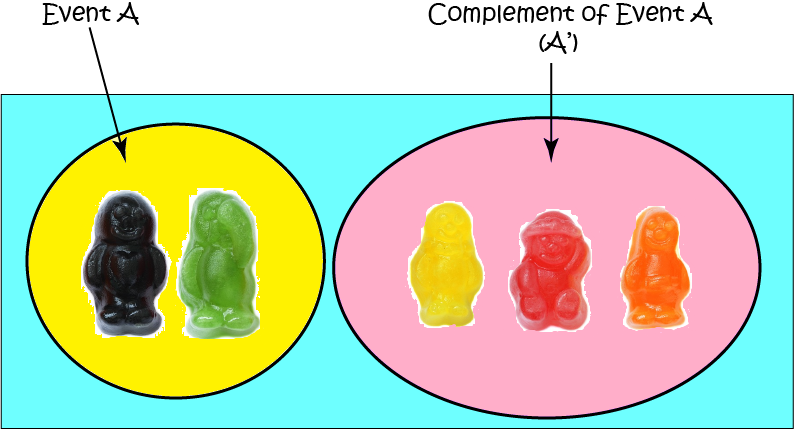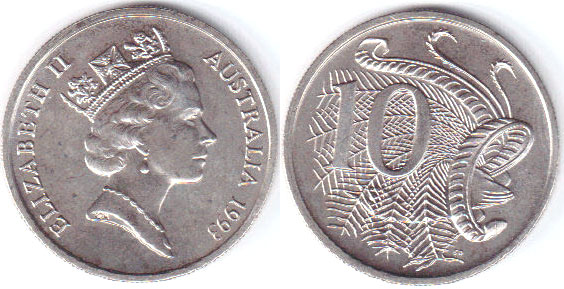Pull Down & Release to Refresh

# Probability: Complement

The complement of an event is a list of all the ways that event doesn't happen. So, it's the list of all outcomes of an experiment that do not form part of that event. Let's look at some examples.

## ExamplesIf the event is $\{\text{Green}\}$, the complement is $\{\text{Red}\}$.If the event is roll an even number $\{2,4,6\}$, the complement is rolling an odd number $\{1,3,5\}$.If the event is drawing a $\{\text{Spade}\}$ from a standard deck of $52$ playing cards, the complement is drawing one of $\{\text{Heart, Diamond, Club}\}$.

If you take an event and its complement together, you get all of the possible outcomes for an experiment.

## Probability

The probability that an event (E) occurs is given by the formula:

$P(E) = \dfrac{\text{Number of Ways the Event E Can Happen}}{\text{Total Number of Outcomes}}$

### Example

What is the probability of rolling an even number on a 6-sided die?

Number of Ways the Event Can Happen: You can roll 2, 4 or 6, so there are 3 ways this can happen.

Total Number of Outcomes: You could roll 1, 2, 3, 4, 5 or 6, so there are 6 possible outcomes.

$\text{Probability} = \dfrac{3}{6} = \dfrac{1}{2}$.

We use $E'$ or $E^c$ to denote the complement of the event $E$ (or $A'$ to denote the complement of event $A$). We always, always have:

$P(E)+ P(E') = 1.$

### ExampleSam's dad loves black and green jelly babies. Event $A$ is going to denote randomly drawing a black or green jelly baby from a bag of jelly babies with equal numbers of the five different colours: green, orange, black, red and yellow. So $A = \{\text{black}, \text{green}\}$ and $A' = \{\text{orange}, \text{red}, \text{yellow}\}$.

The number of ways Event $A$ can occur is $2$, and there are a total of $5$ possible outcomes. So, the probability of event $A$ is:

$P(A) = \dfrac{2}{5}.$

The number of ways the complement of Event $A$ can occur is $3$. As the total number of outcomes is $5$, the probability of event $A'$ is:

$P(A') = \dfrac{3}{5}.$

Let's just check:

$P(A) + P(A') = \dfrac{2}{5} + \dfrac{3}{5} = \dfrac{5}{5} = 1$
Looking good!

## What is the Complement Good For?

Sometimes, it is just easier to work out the probability of the complement of an event and subtract it from $1$.

### ExampleIf you toss a coin three times, what is the probability of getting at least one head?

Solution:

There are quite a few ways that this event $E$ can occur: $\{HHH,HHT,HTH,THH, \dots, TTH\}$, but only one way $\{TTT\}$ that its complement can occur. Thus, it's easier to work out the probability of the complement occurring and subtract it from one. There are a total of $8$ possible outcomes. So, the probability of the complement is

$P(E') = \dfrac{1}{8},$
and the probability of our event occurring is
$P(E) = 1 - P(E') = 1 - \dfrac{1}{8} = \dfrac{7}{8}.$

### Description

In this mini series, you will learn a bit more on the topic of probability, we will cover topics such as

• Ratios
• Fair dice
• Conditional probability
• Mutually exclusive events

and more

### Audience

Year 10 or higher students

### Learning Objectives

Explore more on the topic of probability

Author: Subject Coach
You must be logged in as Student to ask a Question.